# Intermediate Maths Solutions for Trigonometric Equations Exercise 7(a)

Inter Maths 1A Exercise 7(a) Trigonometric Equations solutions are given.

These solutions are very easy to understand. Study the text book lesson very well.

Observe the problems and solutions in the text book. Try them.

Observe the solutions and try them in your own method.

You can see the text book solutions for

Functions

Exercise 1(a)

Exercise 1(b)

Exercise 1(c)

Mathematical Induction

Exercise 2(a)

Matrices

Exercise 3(b)

Exercise 3(c)

Exercise 3(e)

Exercise 3(f)

Exercise 3(g)

Exercise 3(i)

Product of Vectors

Exercise 5(a)

Exercise 5(b)

Trigonometric Ratios upto Transformations

Exercise 6(a)

Exercise 6(b)

Exercise 6(c)

Exercise 6(d)

Exercise 6(e)

Exercise 6(f)

Trigonometric Equations

Exercise 7(a)

Inverse Trigonometric Equations

Exercise 8(a)

Hyperbolic Functions

Exercise 9(a)

Properties of Triangles

Exercise 10(a)

Exercise 10(b)

Inter Maths Trigonometry text book solutions

Maths 1B solutions for

Locus

Transformation of Axes

Exercise 2(a)

Three Dimensional Coordinates

Exercise 5(a)

Exercise 5(b)

Direction Cosines ans Direction Ratios

Exercise 6(a)
Exercise 6(b)
The Plane

Exercise 7(a)

Limits and Continuity

Exercise 8(a)

Exercise 8(b)

Exercise 8(e)

M

# 7(a)

## Maths solutions for Trigonometric Equations Exercise 7(a)

Exercise 7(a)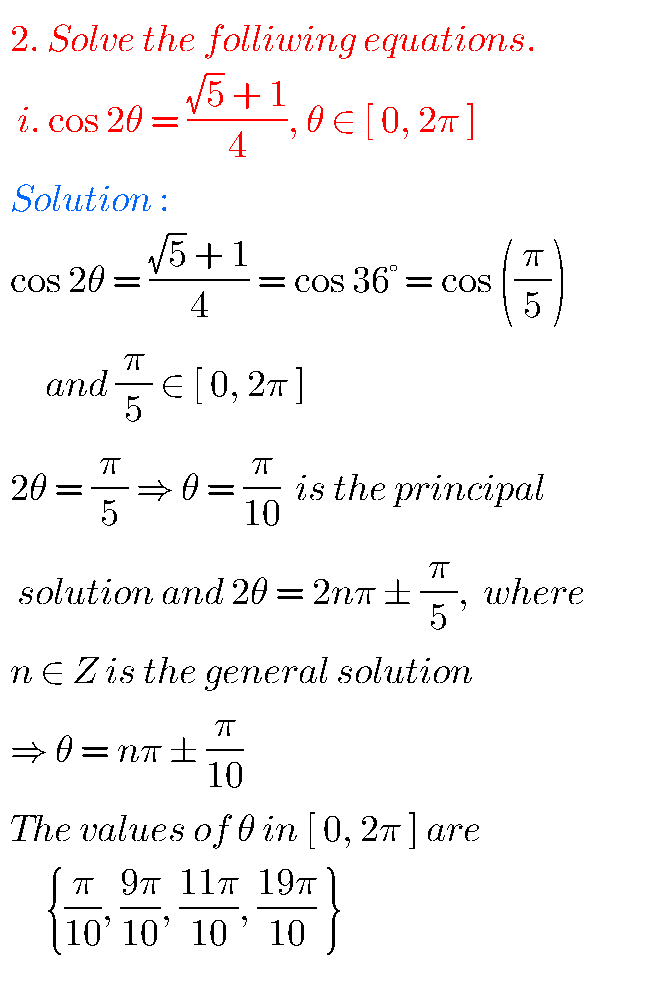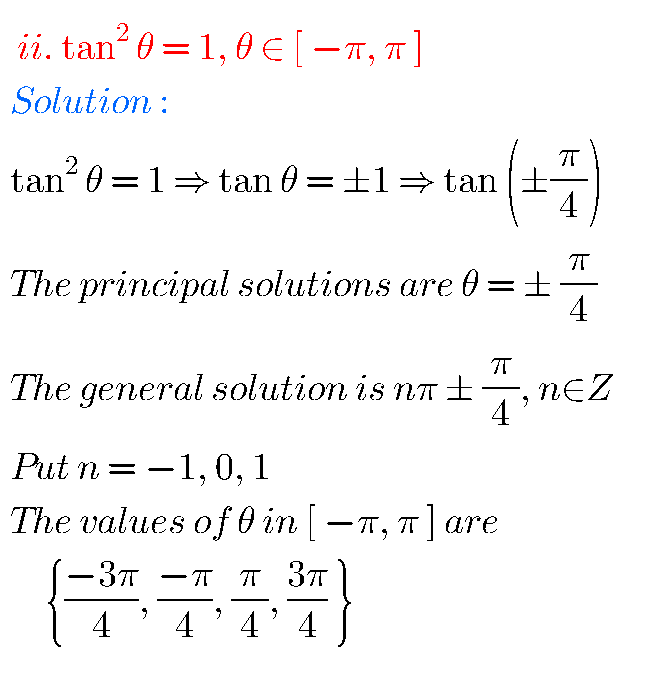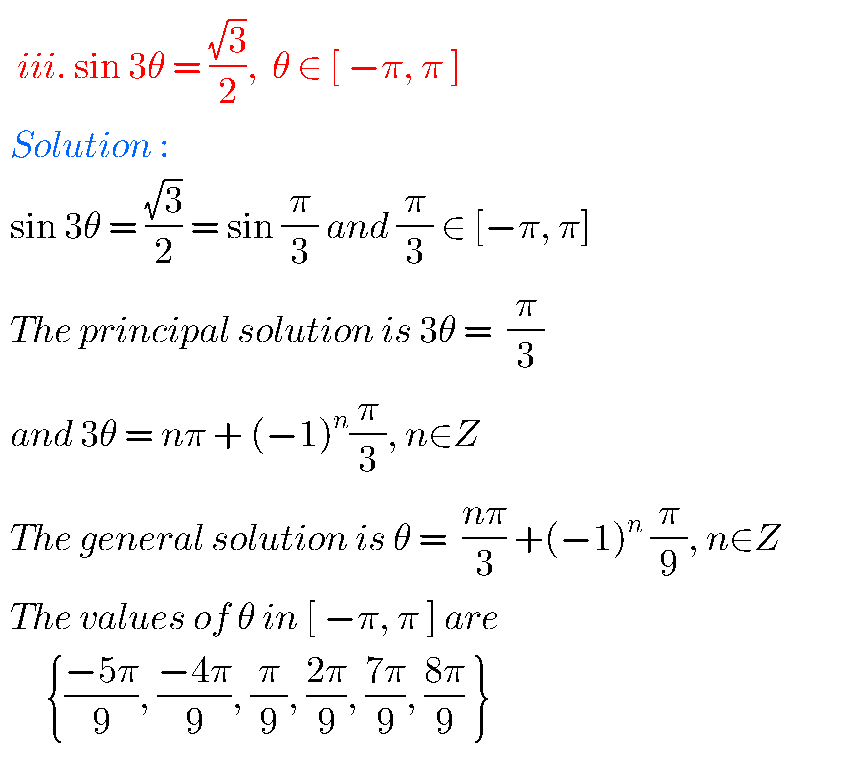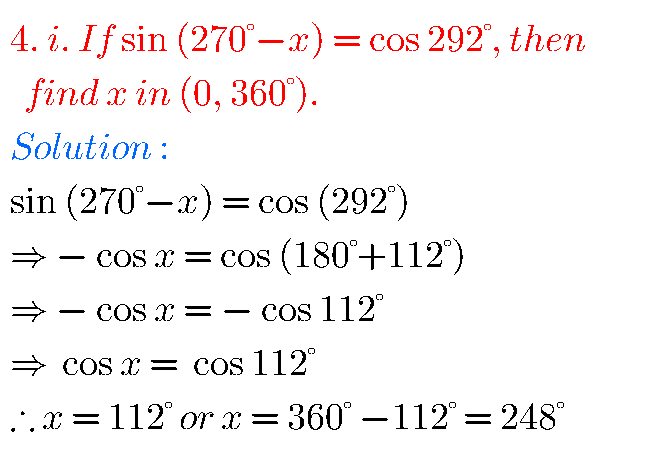## Trigonometric Equations solutions Exercise 7(a) Inter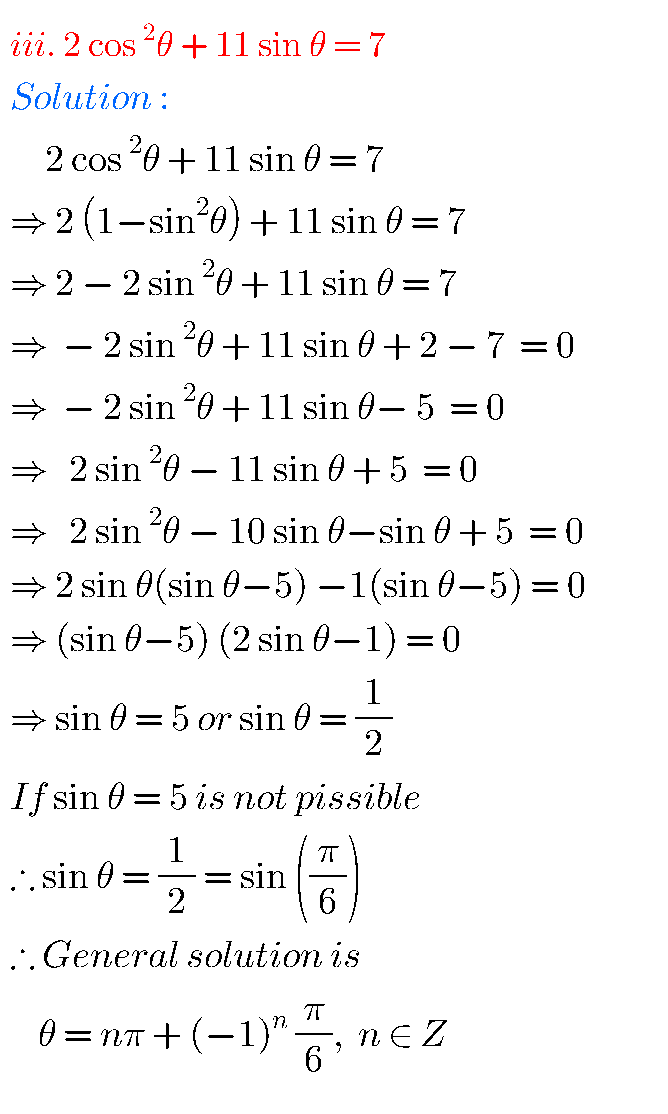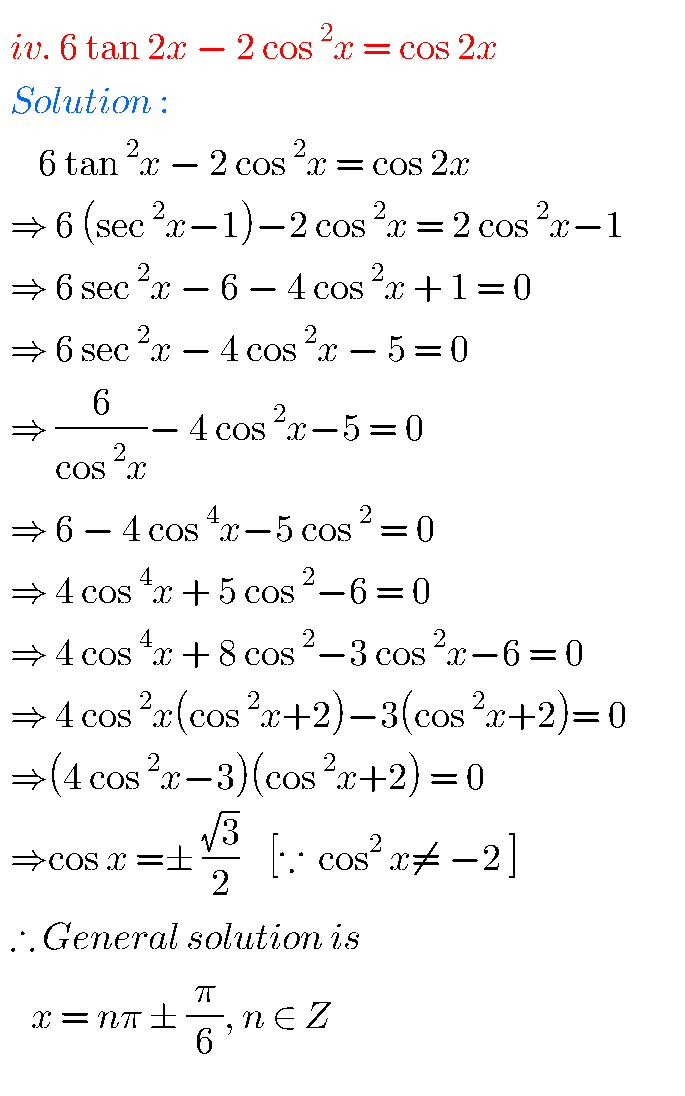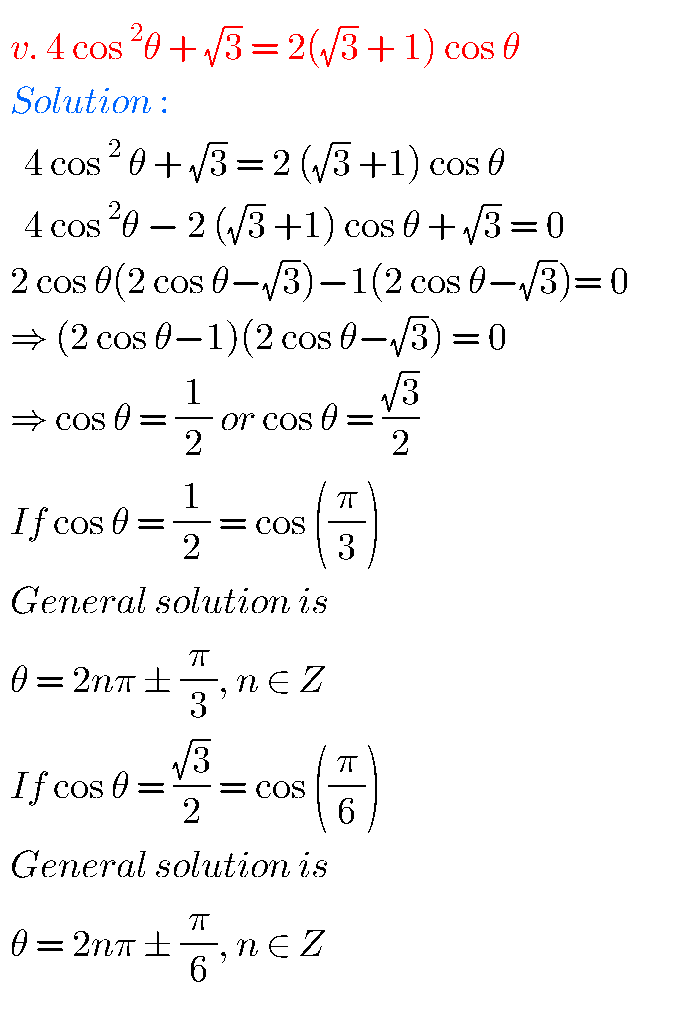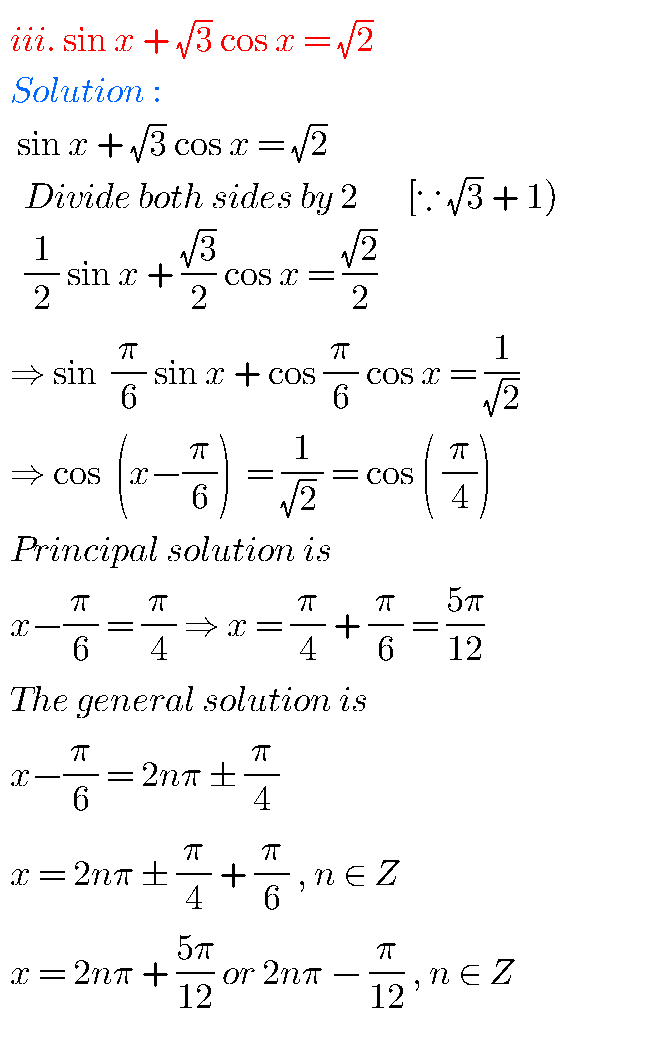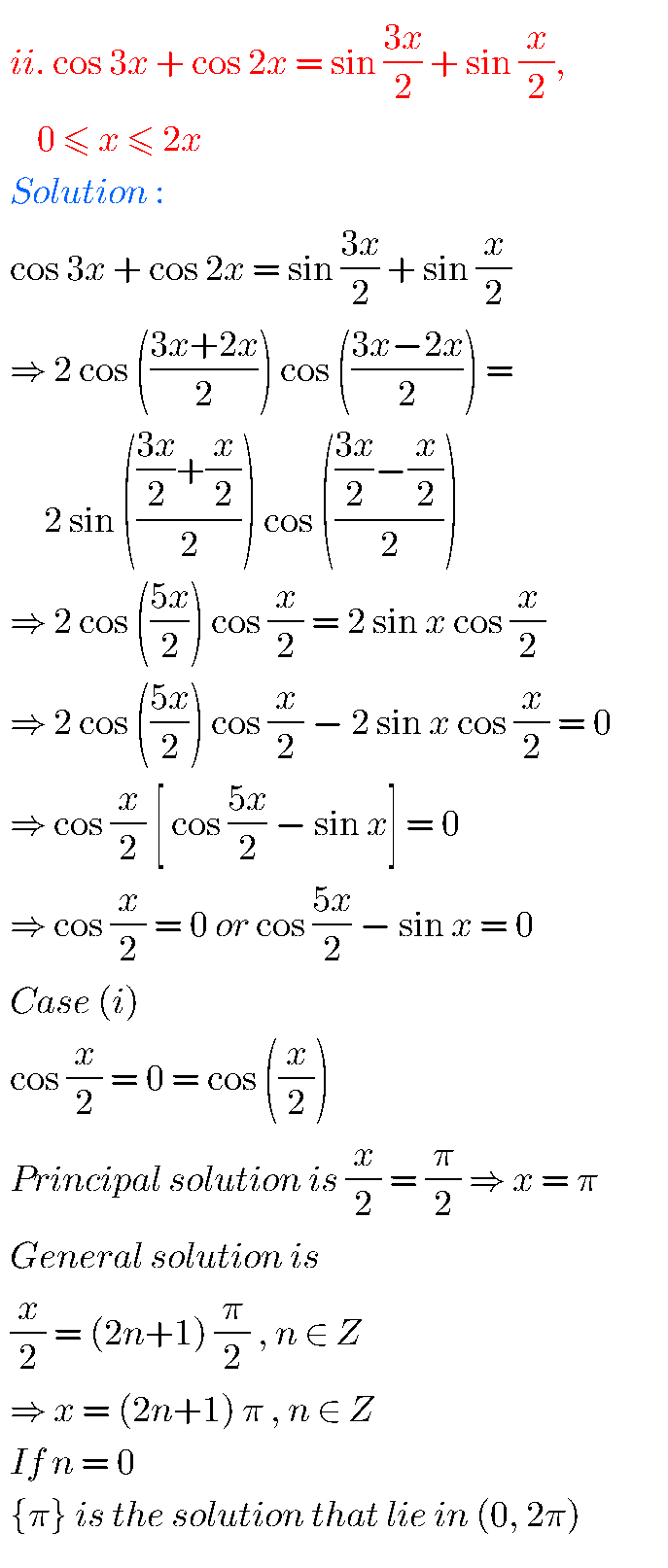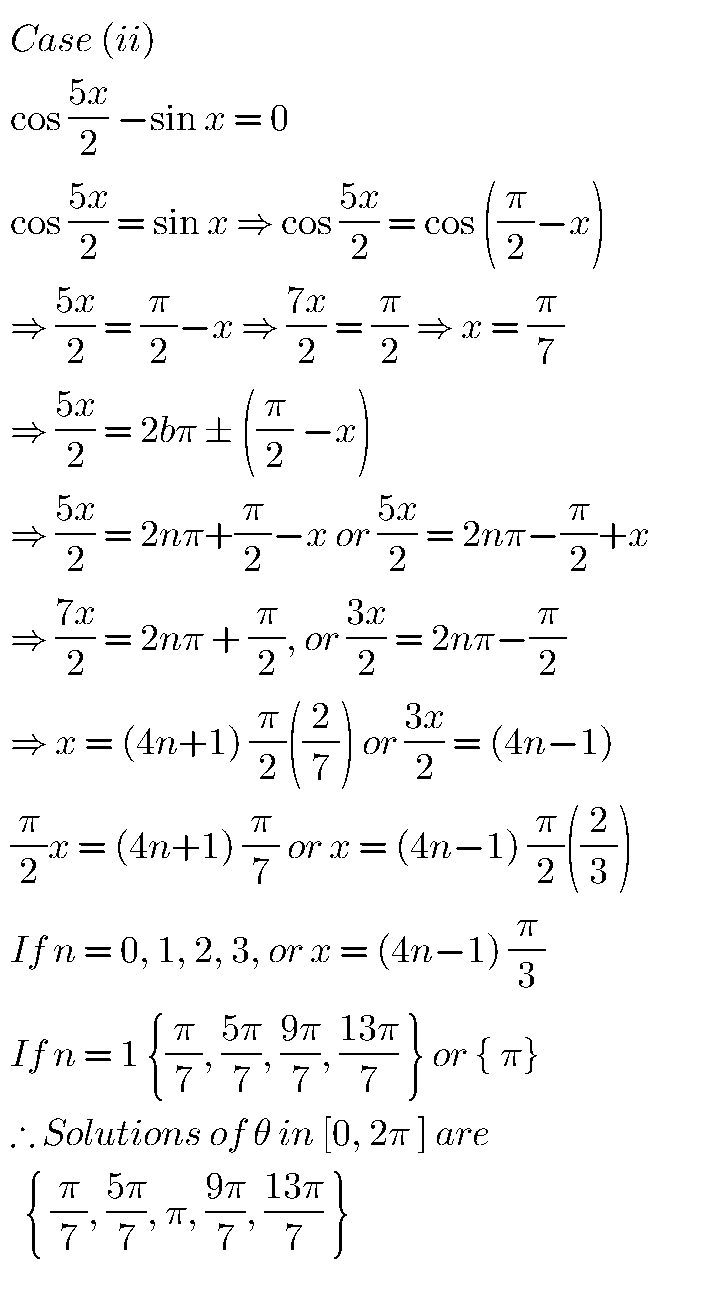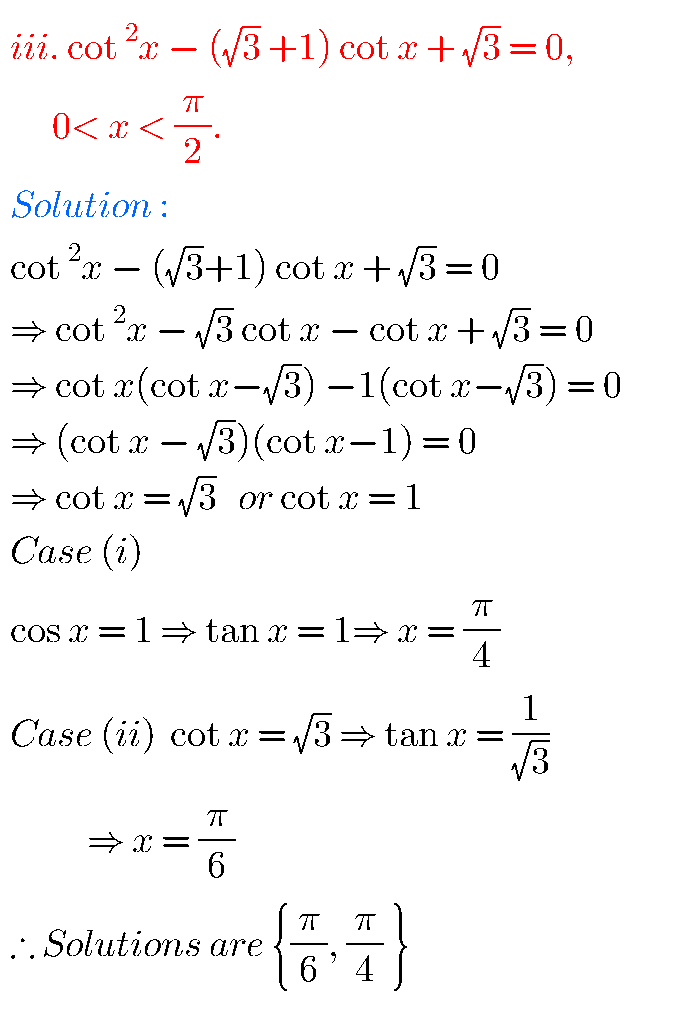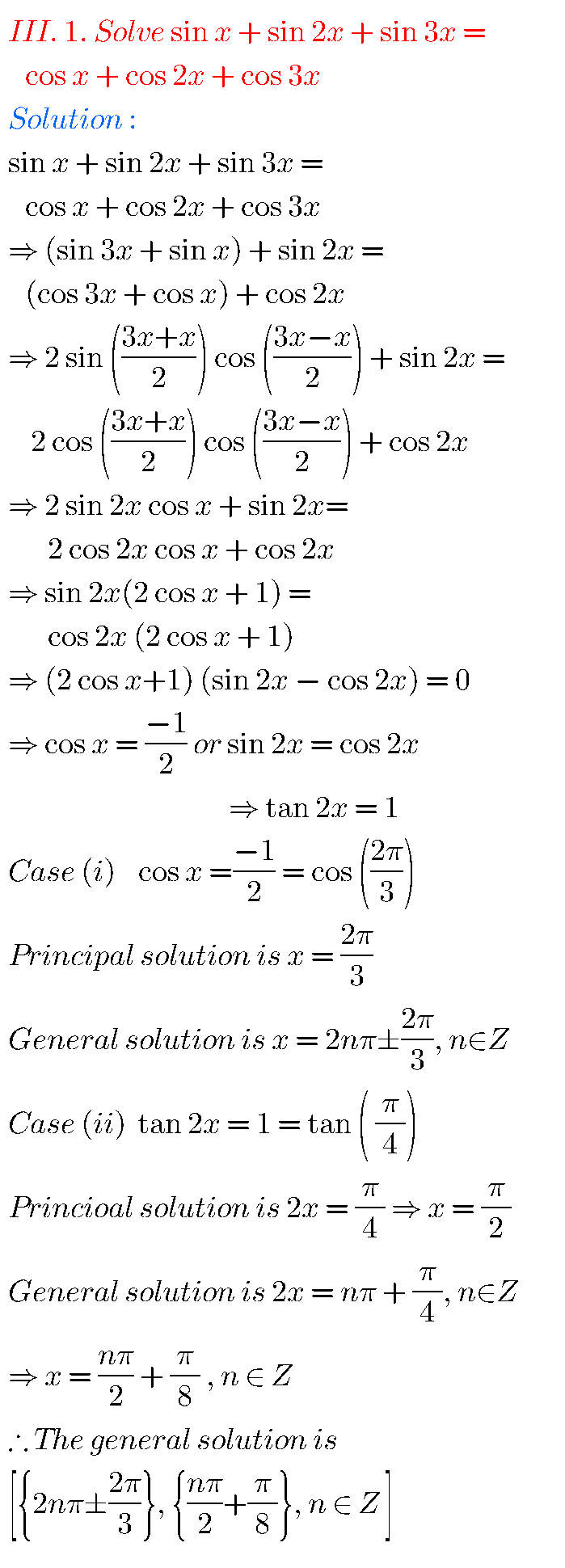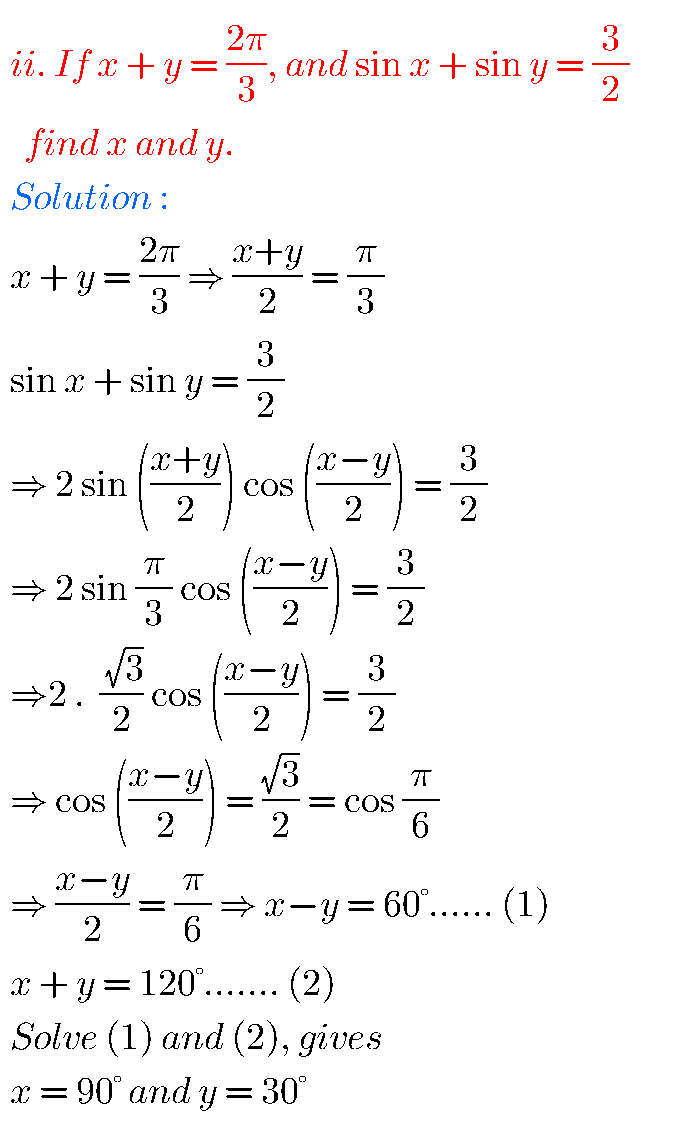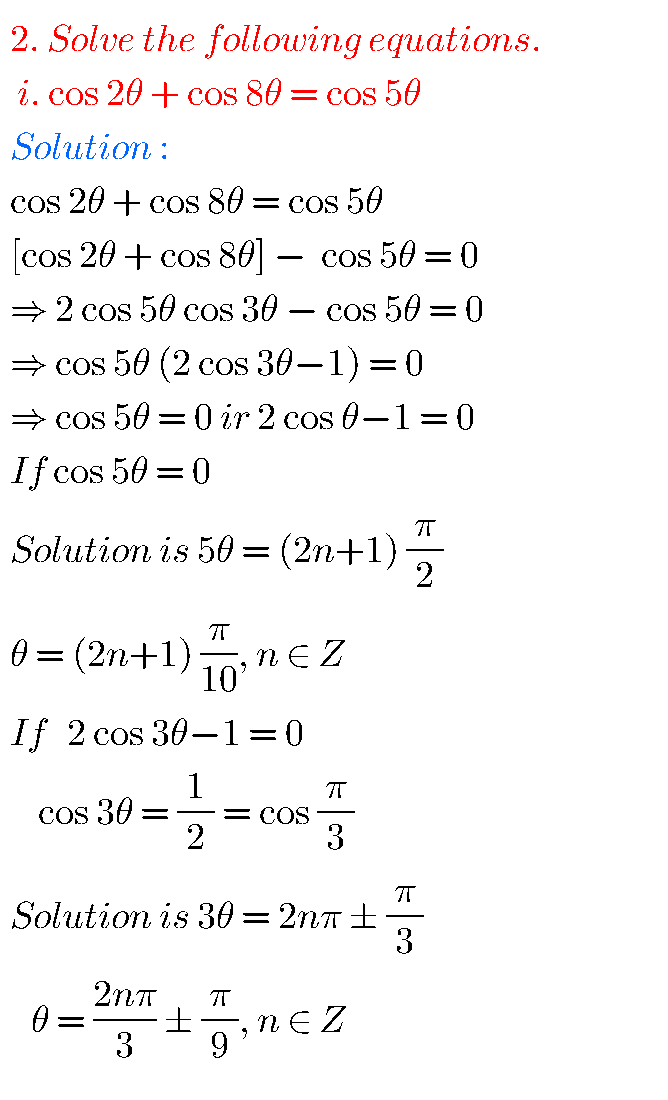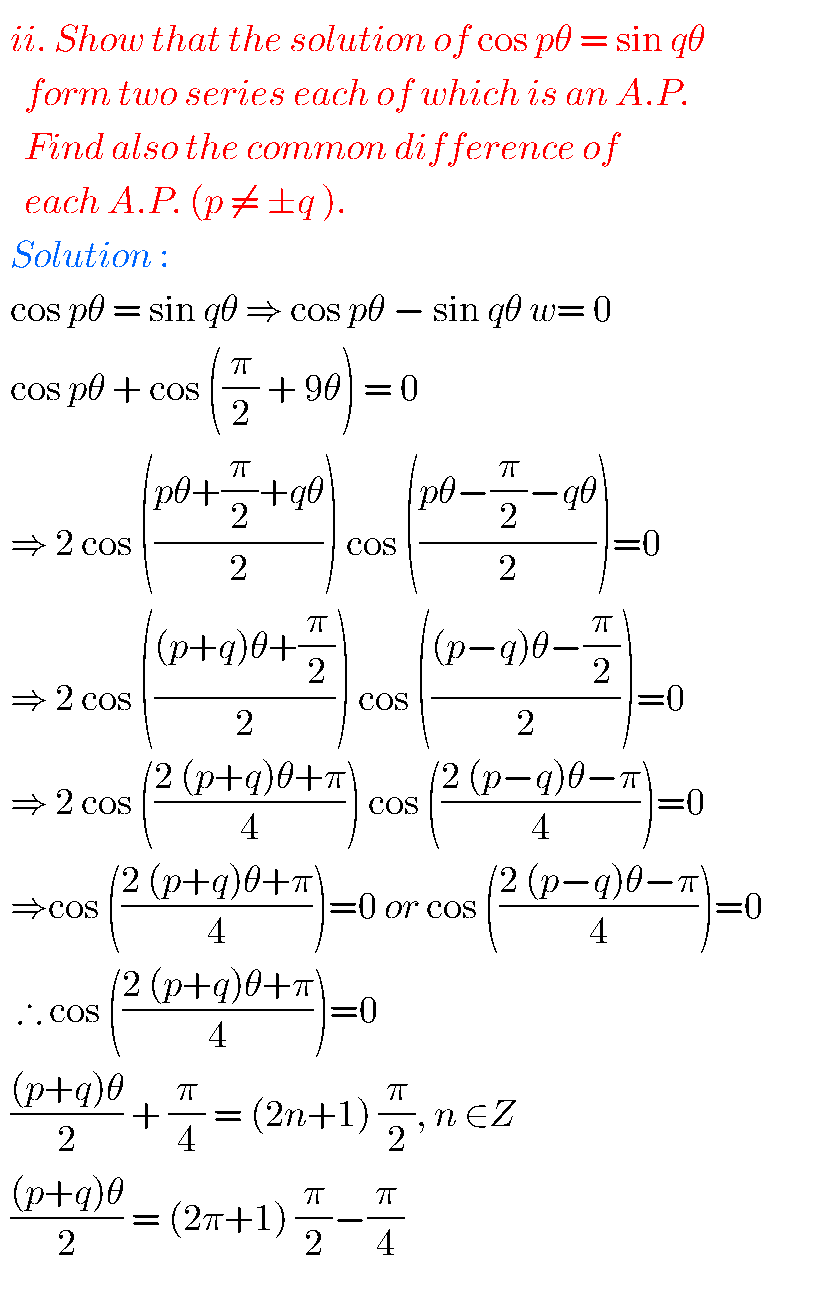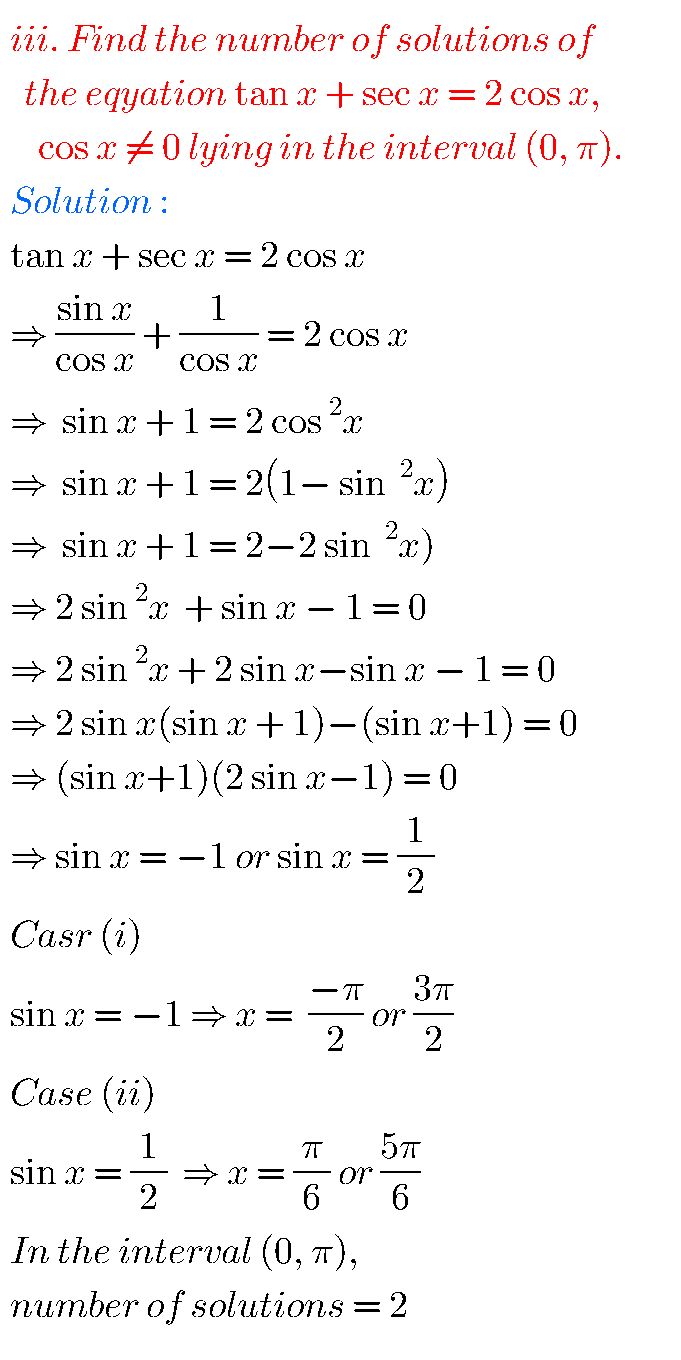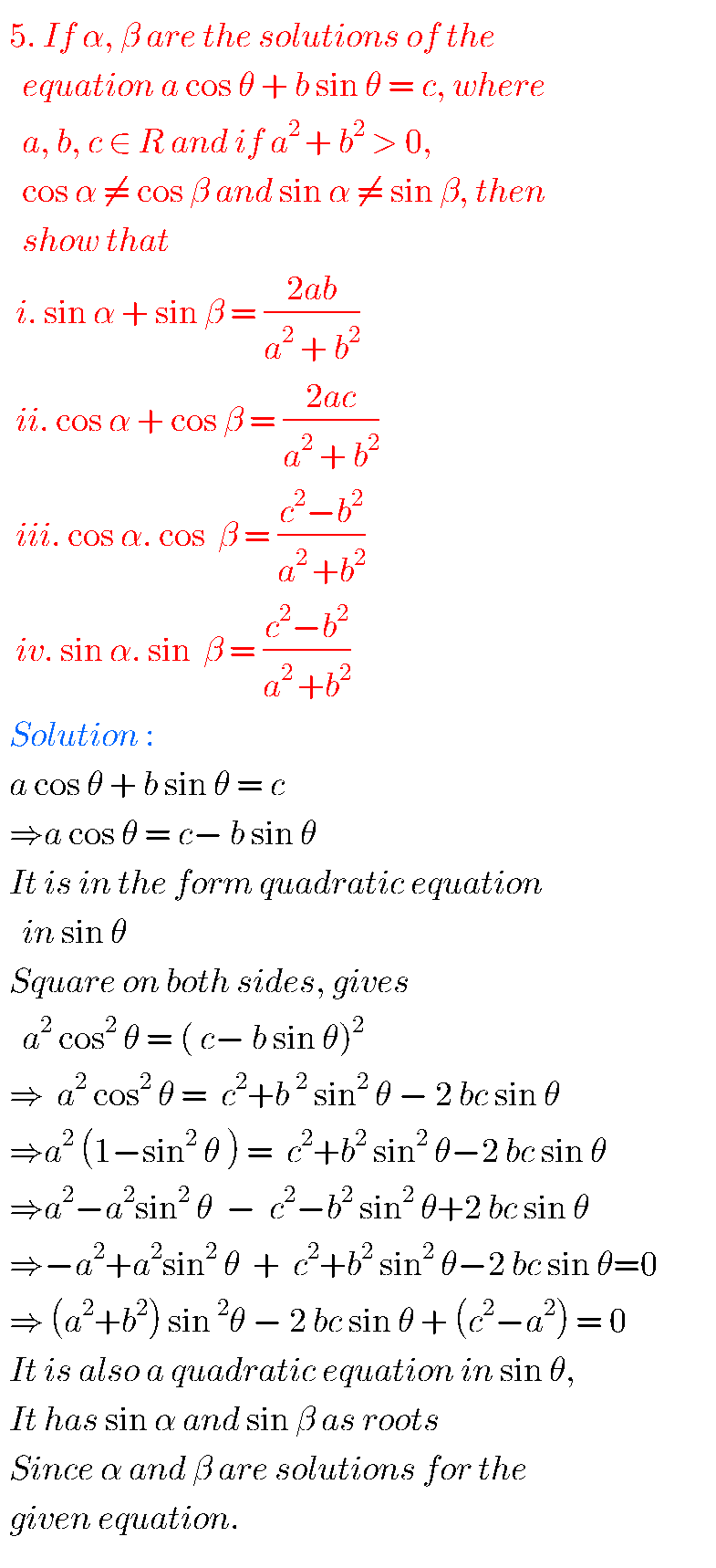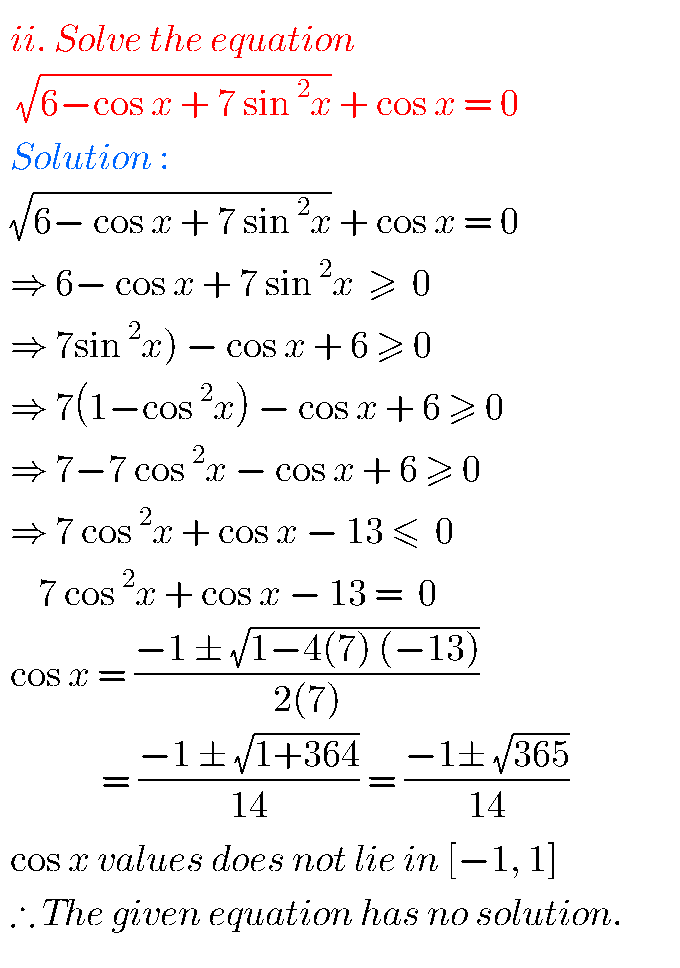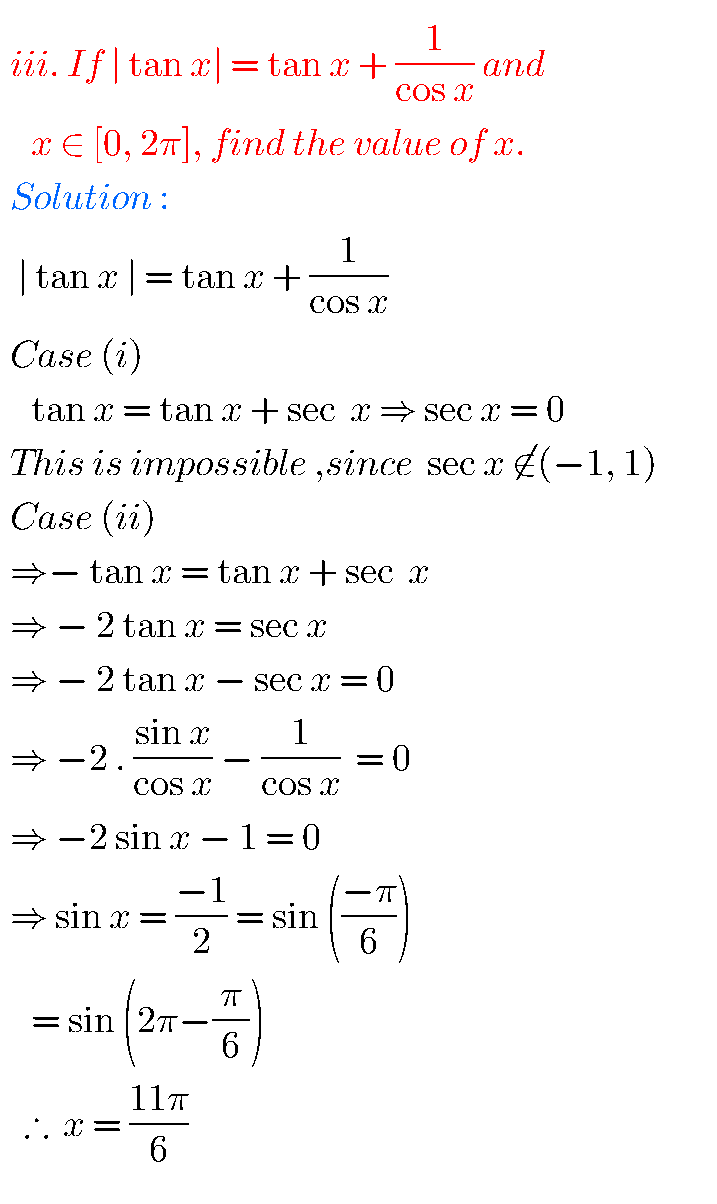Note : Observe the solutions and try them in your own method.

SSC Maths solutions

Inter maths 1A solutions

Inter Maths 1b solutions

Inter Maths IIA text book solutions

Inter Maths IIB text book solutions

You can see the solutions for text book Maths 1A

Functions

Exercise 1(a)

Exercise 1(b)

Exercise 1(c)

Mathematical Induction

Exercise 2(a)

Trigonometric Ratios up to Transformations

Exercise 6(a)

Exercise 6(b)

Exercise 6(c)

Exercise 6(d)

Exercise 6(e)

Exercise 6(f)

Trigonometric Equations

Exercise 7(a)

Inverse Trigonometric Equations

Exercise 8(a)

Hyperbolic Functions

Exercise 9(a)

Properties of Triangles

Exercise 10(a)

Exercise 10(b)

Inter Maths Trigonometry text book solutions

Maths 1B solutions for

Transformation of Axes

Exercise 2(a)

Limits and Continuity

Exercise 8(a)

Exercise 8(b)

M